# 古运河局部河段水质时间变化特性——以运河三湾风景区河段为例Temporal Change Characteristics of Water Quality in a Designated Reach of the Ancient Canal

• 全文下载: PDF(1993KB)    PP.49-61   DOI: 10.12677/AEP.2020.101006
• 下载量: 93  浏览量: 124   国家自然科学基金支持

The main objective of the current research is to reveal the temporal change characteristics of water quality for a designated reach of the Sanwan Scenic Area of Ancient Canal in Yangzhou City. The observation of the water quality parameters such as water temperature (WT), pH, electrical con-ductivity (EC), dissolved oxygen (DO), and concentration of NH4+ had been carried out on the two sections of the main stream and two tributary inflow sections in the period from October 2018 to May 2019. Coefficient of variation method, single factor water quality index method and multiple linear regression method were adopted for data analysis. A flow-following dispersion model for NH4+ calculation was developed and simulation for concentration of NH4+ changed process was carried out in the designated reach. The result indicates that during the study period, the WT sea-sonally changed in each section; the pH and EC represented an upward trend before April 2019 and then decreased; the DO in the tributaries was lower than that in the main stream, but the EC was higher than main stream; the order of the variability and weight coefficient of water quality parameters was DO > WT > EC > pH; the single factor water quality identification index of DO in the downstream was significantly affected by the sewage discharge from the tributaries, the pH and single factor water quality identification index in the tributaries changed randomly. According to results of the multiple linear regression analysis, DO is negatively correlated with WT and pH, the effect of pH change on DO is greater than that of WT; a proper simulation result is achieved for the concentration of NH4+ simulation by using the developed numerical model, and the concentration of NH4+ in the upstream sections decreases gradually, but increases in the downstream sections, the concentration of NH4+ in each section approaches the same level gradually.

1. 引言

2. 材料与方法

2.1. 研究区概况与水质观测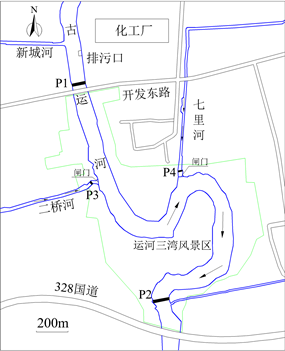Figure 1. Sketch map of the study river reach and the observation sections

2.2. 研究方法

2.2.1. 变异系数法

$V=\frac{S}{\stackrel{¯}{X}}=\frac{\sqrt{\frac{1}{n-1}\underset{i=1}{\overset{n}{\sum }}{\left({X}_{i}-\stackrel{¯}{X}\right)}^{2}}}{\frac{1}{n}\underset{i=1}{\overset{n}{\sum }}{X}_{i}}$ (1)

${A}_{i}=\frac{{V}_{i}}{\underset{i=1}{\overset{n}{\sum }}{V}_{i}}$ (2)

2.2.2. 单因子水质标识指数法

${P}_{i}={X}_{1}\cdot {X}_{2}{X}_{3}$ (3)

X2的计算公式为：

${X}_{2}=\frac{{\rho }_{DOk上}-{\rho }_{DO}}{{\rho }_{DOk上}-{\rho }_{DOk下}}×10$ (4)

${X}_{1}\cdot {X}_{2}=6+\frac{{\rho }_{DO,5下}-{\rho }_{DO}}{{\rho }_{DO,5下}}m$ (5)

m为计算修正系数，一般取4。

X3为水质类别与功能区规划设定类别的比较结果，为一位或两位有效数字。若水质类别好于或达到水环境功能区类别，则X3取0；若水质类别比功能区类别差且X2不为0，则

${X}_{3}={X}_{1}-{f}_{i}$ (6)

${X}_{3}={X}_{1}-{f}_{i-1}$ (7)

2.2.3. 多元线性回归法

$Y=\left[\begin{array}{c}{y}_{1}\\ {y}_{2}\\ {y}_{3}\\ ⋮\\ {y}_{n}\end{array}\right]$$X=\left[\begin{array}{ccc}1& {x}_{11}& {x}_{21}\\ 1& {x}_{12}& {x}_{22}\\ 1& {x}_{13}& {x}_{23}\\ ⋮& ⋮& ⋮\\ 1& {x}_{1n}& {x}_{2n}\end{array}\right]$$B=\left[\begin{array}{c}{b}_{0}\\ {b}_{1}\\ {b}_{2}\end{array}\right]$

${L}_{11}=\underset{i=1}{\overset{n}{\sum }}{\left({X}_{1i}-\stackrel{¯}{{X}_{1}}\right)}^{2}$ (8)

${L}_{12}={L}_{21}=\underset{i=1}{\overset{n}{\sum }}\left({X}_{1i}-\stackrel{¯}{{X}_{1}}\right)\left({X}_{2i}-\stackrel{¯}{{X}_{2}}\right)$ (9)

${L}_{22}=\underset{i=1}{\overset{n}{\sum }}{\left({X}_{2i}-\stackrel{¯}{{X}_{2}}\right)}^{2}$ (10)

${L}_{1y}=\underset{i=1}{\overset{n}{\sum }}\left({X}_{1i}-\stackrel{¯}{{X}_{1}}\right)\left({Y}_{i}-\stackrel{¯}{Y}\right)$ (11)

${L}_{2y}=\underset{i=1}{\overset{n}{\sum }}\left({X}_{2i}-\stackrel{¯}{{X}_{2}}\right)\left({Y}_{i}-\stackrel{¯}{Y}\right)$ (12)

$\left\{\begin{array}{l}{L}_{11}{b}_{1}+{L}_{12}{b}_{2}={L}_{1y}\\ {L}_{21}{b}_{1}+{L}_{22}{b}_{2}={L}_{2y}\end{array}$ (13)

$R=\sqrt{\frac{{b}_{1}{L}_{1y}+{b}_{2}{L}_{2y}}{\underset{i=1}{\overset{n}{\sum }}{y}_{i}^{2}-\frac{1}{n}{\left(\underset{i=1}{\overset{n}{\sum }}{y}_{i}\right)}^{2}}}$ (14)

2.2.4. $N{H}_{4}^{+}$ 运移过程数值模拟

$\frac{\partial h}{\partial t}+\frac{\partial q}{\partial x}=0$ (15)

$\frac{\partial C}{\partial t}+u\frac{\partial C}{\partial x}={K}_{x}\frac{{\partial }^{2}C}{\partial {x}^{2}}-KC$ (16)

${K}_{x}=\alpha h{u}_{\ast }$ (17)

$\alpha =0.011{\left(\frac{W}{h}\right)}^{2}{\left(\frac{u}{{u}_{\ast }}\right)}^{2}$ (18)

${h}_{i}^{n+1}={h}_{i}^{n}-\frac{\Delta t}{\Delta x}\left({q}_{i+1}^{n}-{q}_{i}^{n}\right)$ (19)

${u}_{i}^{n}=\frac{{q}_{i}^{n}}{{h}_{i}^{n}}$ (20)

${C}_{i}^{n+1}={C}_{i}^{n}+\Delta t\left({K}_{x}{}_{i}^{n}\frac{{C}_{i+1}^{n}-2{C}_{i}^{n}+{C}_{i-1}^{n}}{\Delta {x}^{2}}-{u}_{i}^{n}\frac{{C}_{i+1}^{n}-{C}_{i}^{n}}{\Delta x}-K{C}_{i}^{n}\right)$ (21)

$NSE=1-\frac{{\sum }_{t=1}^{T}{\left({Q}_{o}^{t}-{Q}_{m}^{t}\right)}^{2}}{{\sum }_{t=1}^{T}{\left({Q}_{o}^{t}-\stackrel{¯}{{Q}_{o}}\right)}^{2}}$ (22)

3. 结果与分析

3.1. 观测结果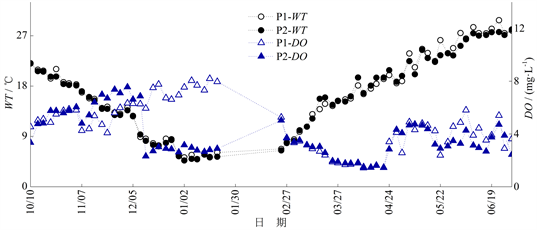Figure 2. The change process of WT and DO in P1 and P2 sections (2018/10/10-2019/6/30, there was no observation in February, 2019)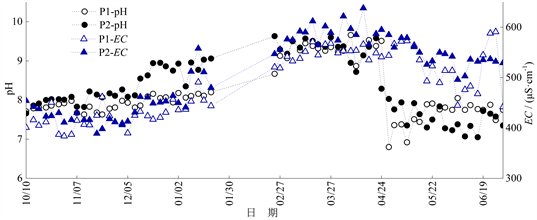Figure 3. The change process of pH and EC in P1 and P2 sections (2018/10/10-2019/6/30, there was no observation in February, 2019)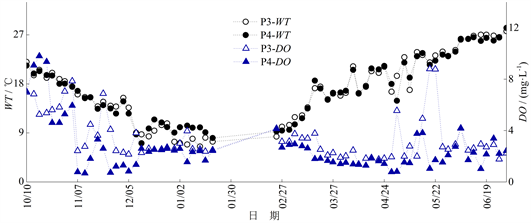Figure 4. The change process of WT and DO in P3 and P4 sections (2018/10/10-2019/6/30, there was no observation in February, 2019)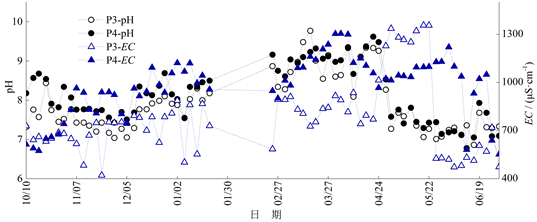Figure 5. The change process of pH and EC in P3 and P4 sections (2018/10/10-2019/6/30, there was no observation in February, 2019)

3.2. 变异性分析Table 1. Quarterly coefficient of variation, overall coefficient of variation and weight of WT and EC in each sectionTable 2. Quarterly coefficient of variation, overall coefficient of variation and weight of DO and pH in each section

3.3. 单因子水质标识指数分析

P1、P2断面以DO为指标的单因子水质标识指数计算结果如图6图7所示。Figure 6. The change process of WT and single factor water quality identification index (Pi) in P1 and P2 sections (2018/10/10-2019/6/30, there was no observation in February, 2019)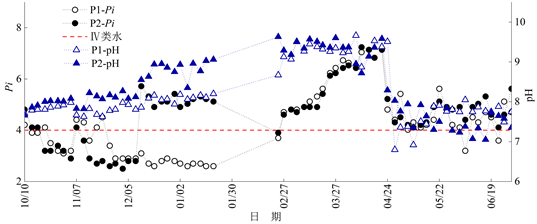Figure 7. The change process of pH and single factor water quality identification index (Pi) in P1 and P2 sections (2018/10/10-2019/6/30, there was no observation in February, 2019)

P3、P4断面以DO为指标的水质标识指数Pi变化过程如图8图9所示。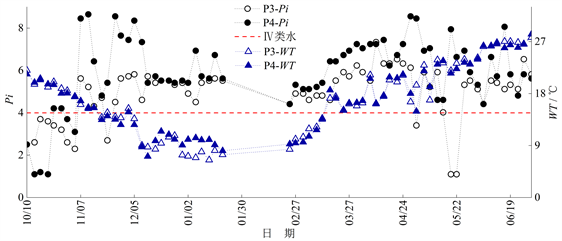Figure 8. The change process of WT and single factor water quality identification index (Pi) in P3 and P4 sections (2018/10/10-2019/6/30, there was no observation in February, 2019)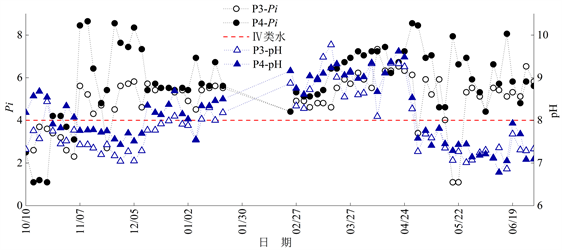Figure 9. The change process of pH and single factor water quality identification index (Pi) in P3 and P4 sections (2018/10/10-2019/6/30, there was no observation in February, 2019)

3.4. 多元线性回归分析结果

P1： $Y=22.461-0.171{X}_{1}-1.833{X}_{2}$ ( $R=0.\text{795}$ )

P2： $Y=18.472-0.108{X}_{1}-1.537{X}_{2}$ ( $R=0.\text{522}$ )

P3： $Y=8.457+0.017{X}_{1}-0.669{X}_{2}$ ( $R=0.\text{3}0\text{1}$ )

P4： $Y=-2.120+0.064{X}_{1}+0.448{X}_{2}$ ( $R=0.203$ )

3.5. $N{H}_{4}^{+}$ 运移过程模拟结果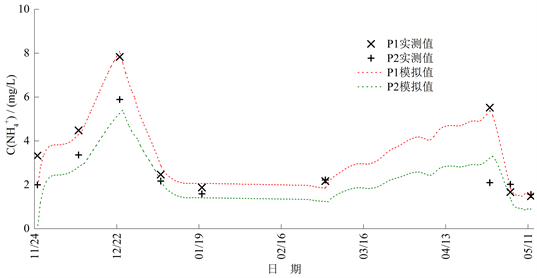Figure 10. The simulated and measured value of concentration of ${\text{NH}}_{4}^{+}$ in P1 and P2 sections (2018/11/25-2019/5/12)

P1断面的纳什效率系数NSE为0.926，P2断面NSE为0.527。P1断面的模拟精度较高，非常接近实测结果，P2断面的模拟精度低于P1断面；大部分时刻P1断面的相对误差均小于10%；2018年12月至2019年1月P2断面相对误差小于20%，模拟精度较高，而2019年3月至5月相对误差均高于30%，最高达到53.5%，其原因是2019年3月至5月WT与河段的流速、水深等水力条件有较大变化，对污染物降解速率产生较大影响，且由于支流排水对P2断面 ${\text{NH}}_{4}^{+}$ 浓度的影响，导致P2断面模拟精度下降。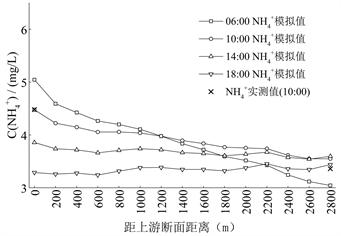(a)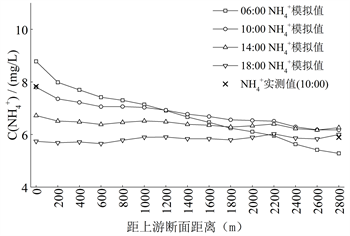(b)

Figure 11. The simulation results of the distribution of ${\text{NH}}_{4}^{+}$ concentration at different times within one day

4. 结论

1) 研究期间WT表现为季节性变化；2019年5月之前研究河段的pH与EC呈上升趋势，之后pH与EC呈下降趋势；大部分时段支流的DO低于干流，EC高于干流。

2) 研究期间各断面常规水质参数的总变异系数与权重从大到小依次为DO > WT > EC > pH；各断面的EC和pH的逐月变异系数基本小于0.1，波动程度较小；大部分时段内P3、P4断面同期的EC、DO、pH的逐月变异系数大于P1、P2。

3) 2018年10月至11月P1、P2断面水质类别多为Ⅲ类水；2018年12月至2019年1月P2断面受支流排水影响导致Pi升高，水质多为Ⅳ、Ⅴ类水；P3、P4断面除2018年10月，其余时段水质类别多为IV、V甚至劣V类水；支流受沿河工业生活污水随机排放影响，pH与Pi变化的随机性较强。

4) 根据P1、P2断面的多元线性回归模型，DO随WT、pH升高而减小，且pH的变化对DO的影响大于WT；P3、P4断面水质由于受沿河工业生活污水随机排放影响较大，因此仅考虑WT和pH这两个因子的多元线性回归模型不能较好地反映三者之间的线性关系。

5) ${\text{NH}}_{4}^{+}$ 随流分散模型对研究河段2018年11月至2019年1月的 ${\text{NH}}_{4}^{+}$ 模拟精度较高，之后时段由于未考虑WT对降解系数K的影响，P2断面的模拟精度较低；单日内河段上游 ${\text{NH}}_{4}^{+}$ 浓度逐渐降低，河段下游 ${\text{NH}}_{4}^{+}$浓度逐渐增大，各断面 ${\text{NH}}_{4}^{+}$ 浓度逐渐趋于同一水平。

NOTES

*通讯作者。

  朱国平, 王秀茹, 王敏, 等. 城市河流的近自然综合治理研究进展[J]. 中国水土保持科学, 2006, 4(1): 92-97.  靳梦, 窦明. 城市化对水系连通功能影响评价研究——以郑州市为例[J]. 中国农村水利水电, 2013(12): 41-45.  Kannel, P.R., Lee, S., Lee, Y.S., Kanel, S.R. and Khan, S.P. (2007) Application of Water Quality Indices and Dissolved Oxygen as Indicators for River Water Classification and Urban Impact Assessment. Environmental Monitoring and Assessment, 132, 93-110.https://doi.org/10.1007/s10661-006-9505-1  Oginni, F.A. (2013) Variations in the Water Quality of an Urban River in Nigeria. Computational Water Energy and Environmental Engineering, 2, 81-91.https://doi.org/10.4236/cweee.2013.22B014  Brion, N., Verbanck, M., Bauwens, W., et al. (2015) Assessing the Impacts of Wastewater Treatment Implementation on the Water Quality of a Small Urban River over the Past 40 Years. En-vironmental Science and Pollution Research, 22, 12720-12736.https://doi.org/10.1007/s11356-015-4493-8  Gliñska-Lewczuk, K., Golaš, I., Koc, J., et al. (2016) The Impact of Urban Areas on the Water Quality Gradient along a Lowland River. Environmental Monitoring and Assessment, 188, 624.https://doi.org/10.1007/s10661-016-5638-z  李如忠, 王超, 汪家权, 等. 基于未确知信息的河流水质模拟预测研究[J]. 水科学进展, 2004, 15(1): 35-39.  武周虎. 河流移流离散水质模型的简化和分类判别条件分析[J]. 水利学报, 2009, 40(1): 27-32.  刘悦忆, 赵建世, 黄跃飞, 等. 基于蒙特卡洛模拟的水质概率预报模型[J]. 水利学报, 2015, 46(1): 51-57.  李强, 范慧芳. 扬州运河水上生态旅游体验产品开发研究[J]. 生态科学, 2016, 35(6): 171-175.  杜娟娟. 基于不同赋权方法的模糊综合水质评价研究[J]. 人民黄河, 2015, 37(12): 69-73.  赵宏, 马立彦, 贾青. 基于变异系数法的灰色关联分析模型及其应用[J]. 黑龙江水利科技, 2007, 35(2): 26-27.  徐祖信. 我国河流单因子水质标识指数评价方法研究[J]. 同济大学学报(自然科学版), 2005, 33(3): 321-325.  毛飞剑, 何义亮, 徐智敏, 等. 基于单因子水质标识指数法的东江河源段水质评价[J]. 安全与环境学报, 2014, 14(5): 327-331.  殷燕, 吴志旭, 刘明亮, 等. 千岛湖溶解氧的动态分布特征及其影响因素分析[J]. 环境科学, 2014, 35(7): 2539-2546.  张景阳, 潘光友. 多元线性回归与BP神经网络预测模型对比与运用研究[J]. 昆明理工大学学报(自然科学版), 2013, 38(6): 61-67.  俞焰, 刘德富, 杨正健, 等. 千岛湖溶解氧与浮游植物垂向分层特征及其影响因素[J]. 环境科学, 2017, 38(4): 1393-1402.  顾莉, 华祖林. 天然河流纵向离散系数确定方法的研究进展[J]. 水利水电科技进展, 2007, 27(2): 85-89.  王瑞, 代丹, 张弛, 等. 太湖不同介质电导率时空变化特征[J/OL]. 环境科学, 2019(10): 1-13.  王有乐, 周智芳, 王立京, 等. 黄河兰州段氨氮降解系数的测定[J]. 兰州理工大学学报, 2006, 32(5): 72-74.